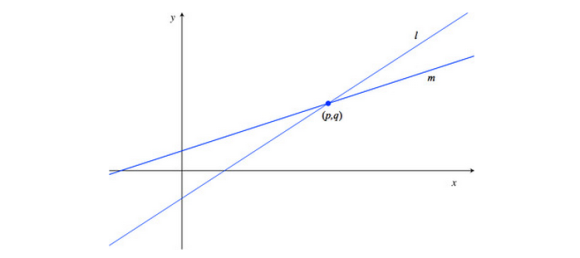• 09/08/13   |   Adjusted: 08/01/18   |   1 file# Equations of Lines

Author: Illustrative Mathematics

• Description
• Files

Mathematically:

• Addresses standards:  8.EE.BMP.1,  MP.3, and MP.6
• Presents an unfamiliar problem that students must make sense of (MP.1)
• Keys directly on the meanings of the parameters in a linear equation as found in cluster 8.EE.B
• Involves two basic forms for the equation of a line (not just the form $y=mx+b$ but also the form $ax+by=c$)

In the classroom:

• Encourages students to share their developing thinking
• Allows students to use precise mathematical language in their arguments (MP.3, MP.6)

This task was designed to include specific features that support access for all students and align to best practice for English Language Learner (ELL) instruction. Go here to learn more about the research behind these supports. This lesson aligns to ELL best practice in the following ways:

• Provides opportunities for students to practice and refine their use of mathematical language.
• Allows for whole class, small group, and paired discussion for the purpose of practicing with mathematical concepts and language.
• Elicits evidence of student thinking both verbally and in written form.
• Includes a mathematical routine that reflects best practices to supporting ELLs in accessing mathematical concepts.
• Provides opportunities to support students in connecting mathematical language with mathematical representations.

• Making the Shifts

How does this task exemplify the instructional Shifts required by CCSSM?

 Focus Belongs to the major work of eighth grade Coherence Builds solid foundation for high school algebra Rigor Conceptual Understanding: primary in this task Procedural Skill and Fluency: secondary in this task Application: not targeted in this task

The figure below shows two lines. One is described by the equation $4x-y=c$ and the other by equation $y=2x+d$, for some constants $c$ and $d$. They intersect at the point $(p, q)$.a. How can you interpret $c$  and $d$ in terms of the graphs of the equations above?

b. Imagine you place the tip of your pencil at point $(p, q)$ and trace line $l$ out of the point with $x$-coordinate $p+2$. Imagine I do the same on line $m$. How much greater would the $y$-coordinate of your ending point be than mine?

• Illustrative Mathematics Commentary and Solution

Commentary:

This task requires students to use the fact that on the graph of the linear equation $y=ax+c$, the $y$-coordinate increases by $a$ when $x$ increases by one. Specific values for $c$ and $d$ were left out intentionally to encourage students to use the above fact as opposed to computing the point of intersection $(p, q)$, and then computing respective function values to answer the question.

Solution:

1. If we put the equation $4x-y=c$ in the form $y=4x-c$, we see that the graph has slope $4$. The slope of the graph of $y=2x+d$ is $2$. So the steeper line, $l$, is the one with equation $y=4x-c$, and therefore $-c$ is the $y$-coordinate of the point where $l$ intersects the $y$-axis. The other line, $m$, is the one with equation $y=2x+d$, so $d$ is the $y$-coordinate of the point where $m$ intersects the $y$-axis.
2. The line $l$ has slope $4$. So on $l$, each increase of one unit in the $x$-value produces an increase of $4$ units in the $y$-value. Thus an increase of $2$ units in the $x$-value produce an increase of $2⋅4=8$ units in the $y$-value. The line $m$ has slope $2$. So on $L_2$, each increase of $1$ unit in the $x$-value produces an increase of $2$ units in the $y$-value. Thus an increase of $2$ units in the $x$-value produces an increase of $2⋅2=4$ units in the $y$-value. Thus your $y$-value would be $8-4=4$ units larger than my $y$-value.
This task related directly to the cluster heading 8.EE.B, "Understand the connections between proportional relationships, lines, and linear equations." Specifically, for each 1 unit moved horizontally, a point moving along the line $y=ax+b$ moves $a$ units vertically. Notice how this sentence involves a proportional relationship, a line in the plane, and the equation of that line.